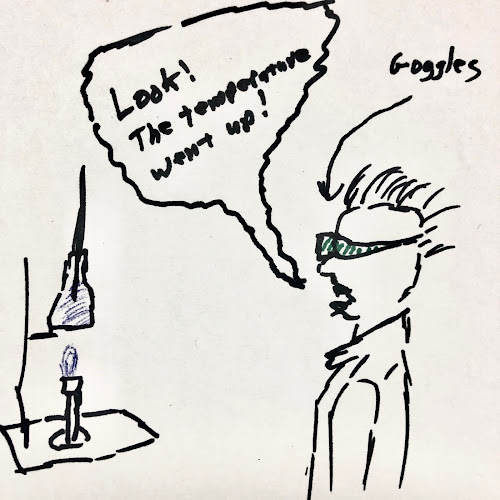## Wednesday, July 29, 2020

### Temperature, Kinetic Energy, and Molecular Motion

Where are we going with this?
The point of this deck is to provide concepts,background information and examples related to the kinetic theory of matter. Using this model will help predict behaviors of substances within a variety of environments and help quantify

Temperature, Kinetic Energy, and Molecular Motion

Let me just apologize on the front end… This concept spirals in on itself. And drag some other concepts into the vortex with it.

Let's jump into the shallow end first… Matter is made of particles. Hold that thought…

Okay, what else is easy? Everyone recognizes the concept of temperature. We ask things like, "How hot is it outside?" We say, "Wow! It's cold in here!"
Maybe no one actually says "wow" but…

So, the concept of temperature is intuitive, to some degree. Hold that thought…

Let's drag in the concept of energy into this spiral… Energy is a quantity that is the potential to do work, which is yet another concept—but we're going to skip over that… Back to energy…

Three are different ways to store energy. Energy can change forms, too, so (example) chemical energy stored in a car battery can be converted to electrical energy.

We're getting back to kinetic theory, now. One way to store energy (a type of energy) is thermal energy

Thermal… that sounds familiar?

Thermal energy is the energy that is stored within the motion of the particles that make up matter.

We're close now!

This brings us to the concept of temperature.

Temperature is a basic measurement that is related to the amount of thermal energy stored in a material. This energy is stored in the motion of the particles. As thermal energy is added, the molecules (let's say we're talking about a solid, and let's use a word we can visualize) vibrate within their relative position to other particles of the substance.

The amount of energy stored is a function of how fast the particles are moving (let's just keep thinking of them vibrating in place, for now) and how much mass each particle has. Of course there is a formula for energy associated with a moving object:

Ek = 1/2mv2
where Ek is kinetic energy, m is mass and v is velocity.

Thermal energy is the sum of all the kinetic energy of all particles of a substance.

This, at last, brings us to a definition of temperature:

Temperature is the average kinetic energy of the molecules in a sample.

So, temperature can be understood as the average kinetic energy of the molecules as they move in constant motion.

Now for the spiral!

If the velocity of the particles goes up, the the kinetic energy goes up (see the equation above). That means that the temperature goes up (since temperature is the average kinetic energy.

But, since we can't see the particles speeding up or slowing down, we generally rely on the temperature to tell is what they are doing.

We can measure the temperature of the water and say, "Well look! The temperature went up."

If that happens, then we can conclude that the particles are moving faster. If the temperature goes down, they are moving slower.

 Temperature Up Temperature Down Kinetic Energy Increases (by definition) Kinetic Energy Decreases (by definition) Particle velocity Increases (according to formula) Particle velocity Decreases (according to formula) Volume Usually Increases Volume Usually DecreasesNot produced by art major.